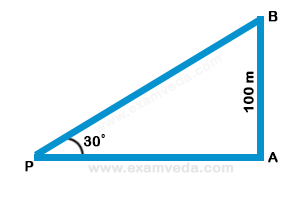# From a point P on a level ground, the angle of elevation of the top tower is 30º. If the tower is 100 m high, the distance of point P from the foot of the tower is:

A. 149 m

B. 156 m

C. 173 m

D. 200 m$$\frac{{AB}}{{AP}} = \tan {30^ \circ } = \frac{1}{{\sqrt 3 }}$$